Search

About 22 Search Results Matching Types of Worksheet, Worksheet Section, Generator, Generator Section, Subjects matching Geometry, Similar to Reading a Protractor Worksheet 2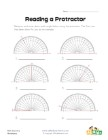Reading a Protractor Worksheet 2

Read each protractor and write down the angles the...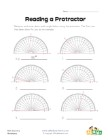Reading a Protractor Worksheet 1

Read each protractor and write down the angles the...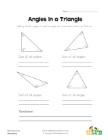Angles in a Triangle Worksheet

Kids learn that the angles of a triangle always ad...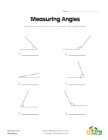Measuring Angles Worksheet 1

Measure each of the angles with a protractor and w...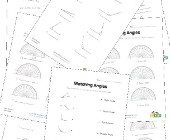Angles Worksheets

Help kids learn about angles with this collection ...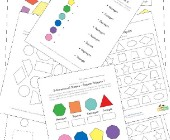2 Dimensional Shapes Worksheets

Check out our collection of 2D shapes worksheets f...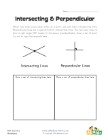Intersecting and Perpendicular Lines Worksheet

Learn about intersecting and perpendicular lines.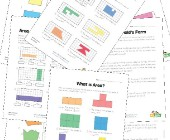Area and Perimeter Worksheets

Help kids learn to calculate the area and perimete...